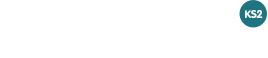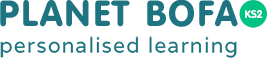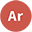####Arithmetic GO TO DEMO

#### Information

What do the Arithmetic tests include?

The tests contain 5400 questions, covering all the syllabus of the NEW Key Stage 2 National Curriculum for Mathematics for Arithmetic.

The tests are designed to be similar to the Key Stage 2 Mathematics Arithmetic Tests.

Tips

Watch the video to see how the platform works.

Do the Arithmetic Demo test.

Some Tips before you start...
• Work through the initial test on your own.
• You can then get help going through the explanations and similar practice questions.
• Wait as long as possible before doing the retest.
• Work through the retest, which contains similar questions to your errors on the initial test.
• You can then see the errors from the retest in the report.
Arithmetic Test Structure

15 minutes

15 questions in each test

4 Test Levels
Level 3
30 tests
Arithmetic questions for Year 3 & 4
Level 4
30 tests
Arithmetic questions for Year 4 & 5
Level 5
30 tests
Arithmetic questions for Year 5 & 6
Level 6
30 tests
Arithmetic questions for Year 6
Information for pupils

The tests are designed to be done, one per week, after a pupil has covered all the material at each level in school.

Try and use as large a screen as possible.

We recommend you use pen/pencil and paper.

BOFA® will assess understanding and fill in any gaps in your knowledge.

Want to know more?

Watch the video to hear from some of our schools.

Do the Arithmetic Demo test.

Find out more with our How it Works Guide.

#### Arithmetic Level 5 Tests View Reports / Preview Tests

What do the buttons do?

Start a new test.

Continue a test you've already started. You might not have finished the practice or re-test stage.

Retake a test you have previously taken. This will delete the previous test data and start from scratch.

View the questions you got wrong at the end of the re-test.

Scores
Test Date Time spent Initial Retest Out of Time Final score Options
Arithmetic Level 5 Test 1
Arithmetic Level 5 Test 2
Arithmetic Level 5 Test 3
Arithmetic Level 5 Test 4
Arithmetic Level 5 Test 5
Arithmetic Level 5 Test 6
Arithmetic Level 5 Test 7
Arithmetic Level 5 Test 8
Arithmetic Level 5 Test 9
Arithmetic Level 5 Test 10
Arithmetic Level 5 Test 11
Arithmetic Level 5 Test 12
Arithmetic Level 5 Test 13
Arithmetic Level 5 Test 14
Arithmetic Level 5 Test 15
Arithmetic Level 5 Test 16
Arithmetic Level 5 Test 17
Arithmetic Level 5 Test 18
Arithmetic Level 5 Test 19
Arithmetic Level 5 Test 20
Arithmetic Level 5 Test 21
Arithmetic Level 5 Test 22
Arithmetic Level 5 Test 23
Arithmetic Level 5 Test 24
Arithmetic Level 5 Test 25
Arithmetic Level 5 Test 26
Arithmetic Level 5 Test 27
Arithmetic Level 5 Test 28
Arithmetic Level 5 Test 29
Arithmetic Level 5 Test 30

Test Arithmetic Level 5 Test 1 Start